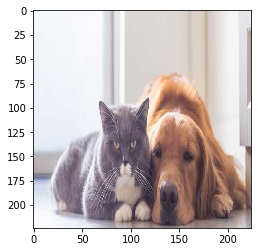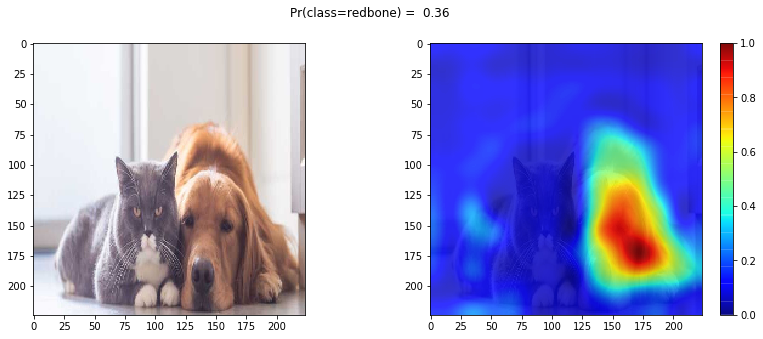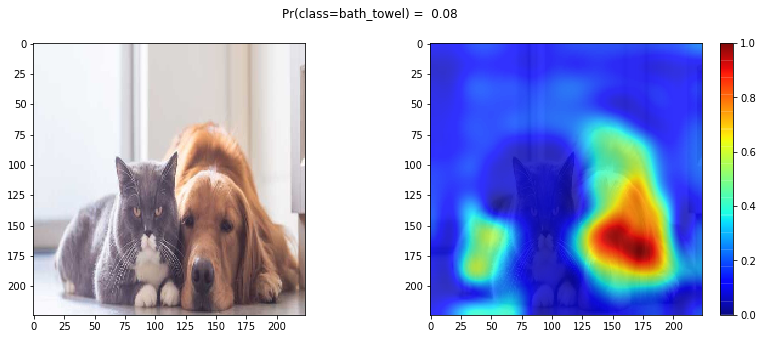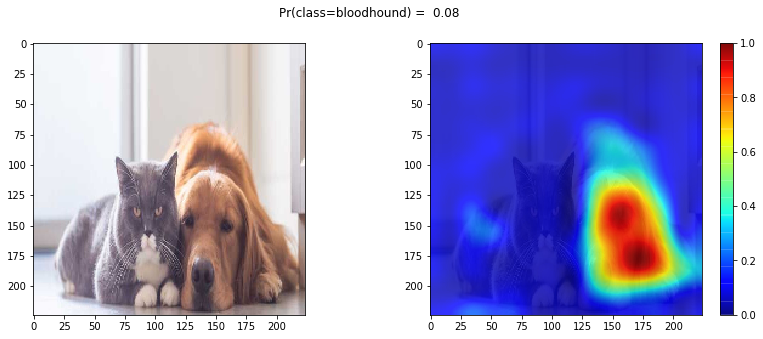# Grad-CAM with keras-vis

Gradient Class Activation Map (Grad-CAM) for a particular category indicates the discriminative image regions used by the CNN to identify that category.

The goal of this blog is to:

• understand concept of Grad-CAM
• understand Grad-CAM is generalization of CAM
• understand how to use it using keras-vis
• implement it using Keras's backend functions.

# Reference¶

## Reference in this blog¶

To set up the same conda environment as mine, follow:

Visualization of deep learning classification model using keras-vis

# Setup¶

In :
import keras
import tensorflow as tf
import vis ## keras-vis
import matplotlib.pyplot as plt
import numpy as np
print("keras      {}".format(keras.__version__))
print("tensorflow {}".format(tf.__version__))

Using TensorFlow backend.

keras      2.2.2
tensorflow 1.10.0


## Read in pre-trained model¶

For this exersize, I will use VGG16.

In :
from keras.applications.vgg16 import VGG16, preprocess_input
model = VGG16(weights='imagenet')
model.summary()
for ilayer, layer in enumerate(model.layers):
print("{:3.0f} {:10}".format(ilayer, layer.name))

_________________________________________________________________
Layer (type)                 Output Shape              Param #
=================================================================
input_1 (InputLayer)         (None, 224, 224, 3)       0
_________________________________________________________________
block1_conv1 (Conv2D)        (None, 224, 224, 64)      1792
_________________________________________________________________
block1_conv2 (Conv2D)        (None, 224, 224, 64)      36928
_________________________________________________________________
block1_pool (MaxPooling2D)   (None, 112, 112, 64)      0
_________________________________________________________________
block2_conv1 (Conv2D)        (None, 112, 112, 128)     73856
_________________________________________________________________
block2_conv2 (Conv2D)        (None, 112, 112, 128)     147584
_________________________________________________________________
block2_pool (MaxPooling2D)   (None, 56, 56, 128)       0
_________________________________________________________________
block3_conv1 (Conv2D)        (None, 56, 56, 256)       295168
_________________________________________________________________
block3_conv2 (Conv2D)        (None, 56, 56, 256)       590080
_________________________________________________________________
block3_conv3 (Conv2D)        (None, 56, 56, 256)       590080
_________________________________________________________________
block3_pool (MaxPooling2D)   (None, 28, 28, 256)       0
_________________________________________________________________
block4_conv1 (Conv2D)        (None, 28, 28, 512)       1180160
_________________________________________________________________
block4_conv2 (Conv2D)        (None, 28, 28, 512)       2359808
_________________________________________________________________
block4_conv3 (Conv2D)        (None, 28, 28, 512)       2359808
_________________________________________________________________
block4_pool (MaxPooling2D)   (None, 14, 14, 512)       0
_________________________________________________________________
block5_conv1 (Conv2D)        (None, 14, 14, 512)       2359808
_________________________________________________________________
block5_conv2 (Conv2D)        (None, 14, 14, 512)       2359808
_________________________________________________________________
block5_conv3 (Conv2D)        (None, 14, 14, 512)       2359808
_________________________________________________________________
block5_pool (MaxPooling2D)   (None, 7, 7, 512)         0
_________________________________________________________________
flatten (Flatten)            (None, 25088)             0
_________________________________________________________________
fc1 (Dense)                  (None, 4096)              102764544
_________________________________________________________________
fc2 (Dense)                  (None, 4096)              16781312
_________________________________________________________________
predictions (Dense)          (None, 1000)              4097000
=================================================================
Total params: 138,357,544
Trainable params: 138,357,544
Non-trainable params: 0
_________________________________________________________________
0 input_1
1 block1_conv1
2 block1_conv2
3 block1_pool
4 block2_conv1
5 block2_conv2
6 block2_pool
7 block3_conv1
8 block3_conv2
9 block3_conv3
10 block3_pool
11 block4_conv1
12 block4_conv2
13 block4_conv3
14 block4_pool
15 block5_conv1
16 block5_conv2
17 block5_conv3
18 block5_pool
19 flatten
20 fc1
21 fc2
22 predictions


Download a json file containing ImageNet class names.

wget "https://raw.githubusercontent.com/raghakot/keras-vis/master/resources/imagenet_class_index.json"

Read in the class index json file

In :
import json
classlabel  = []
for i_dict in range(len(CLASS_INDEX)):
classlabel.append(CLASS_INDEX[str(i_dict)])
print("N of class={}".format(len(classlabel)))

N of class=1000


Let's read in an image that contains both dog and cat. Clearly this image would be very confusing for a model trained with ImageNet, which often has a single object per image.

The goal of this exersise is to understand why VGG16 model makes classification decision.

In :
from keras.preprocessing.image import load_img, img_to_array
plt.imshow(_img)
plt.show()Let's predict the object class of this image, and show the top 5 predicted classes.

Unfortunately, the top 2 predicted class does not make any sense. Why towel?? However, you see that other predicted classes are dogs and kind of makes sense:

Top 1 predicted class:     Pr(Class=redbone            [index=168])=0.360
Top 3 predicted class:     Pr(Class=bloodhound         [index=163])=0.076
Top 4 predicted class:     Pr(Class=basenji            [index=253])=0.042
Top 5 predicted class:     Pr(Class=golden_retriever   [index=207])=0.041
In :
img               = img_to_array(_img)
img               = preprocess_input(img)
y_pred            = model.predict(img[np.newaxis,...])
class_idxs_sorted = np.argsort(y_pred.flatten())[::-1]
topNclass         = 5
for i, idx in enumerate(class_idxs_sorted[:topNclass]):
print("Top {} predicted class:     Pr(Class={:18} [index={}])={:5.3f}".format(
i + 1,classlabel[idx],idx,y_pred[0,idx]))

Top 1 predicted class:     Pr(Class=redbone            [index=168])=0.360
Top 2 predicted class:     Pr(Class=bath_towel         [index=434])=0.076
Top 3 predicted class:     Pr(Class=bloodhound         [index=163])=0.076
Top 4 predicted class:     Pr(Class=basenji            [index=253])=0.042
Top 5 predicted class:     Pr(Class=golden_retriever   [index=207])=0.041


### Last Convolusional Layer of the CNN¶

Let $A^k \in \mathbb{R}^{u\textrm{ x } v}$ be the $k$th feature map ($k=1,\cdots,K$) from the last convolusional layer. The dimension of this feature map is height $u$ and width $v$. For example, in the case of VGG16, $u=14, v=14, K=512$. Grad-CAM utilize these $A^k$ to visualize the decision made by CNN.

#### Some observations¶

• Convolutional feature map retain spatial information (which is lost in fully-connected layers)
• Each kernel represents some visual patterns. For example, one kernel may capture a dog, another kernel may capture bird etc.
• Each pixel of the feature map indicates whether the corresponding kernel's visual pattern exists in its receptive fields.
• Last Convolusional Layer can be thought as the features of a classification model.

$$y^c = f(A^1,...,A^{512})$$

#### Idea¶

• Visualization of the final feature map ($A^k$) will show the discriminative region of the image.
• Simplest summary of all the $A^k,k=1,...,K$ would be its linear combinations with some weights.
• Some feature maps would be more important to make a decision on one class than others, so weights should depend on the class of interest.

$$L^c_{Grad-CAM} \sim = \sum_{k=1}^K \alpha_k^c A^k \in \mathbb{R}^{u\textrm{ x } v}$$

So the question is, what the weights $\alpha_k^c$ should be?

#### Calculating $\alpha_{k}^c$¶

The gradient of the $c$th class score with respect to feature maps $A^{k}$

$$\frac{d y^c}{d A^k_{i,j}}$$

measures linear effect of $(i,j)$th pixel point in the $k$th feature map on the $c$th class score. So averaging pooling of this gradient across $i$ and $j$ explains the effect of feature map $k$ on the $c$th class score.

Grad-CAM propose to use this averaged gradient score as a weights for feature map. $$\alpha_{k}^c = \frac{1}{uv} \sum_{i=1}^u \sum_{j=1}^v \frac{d y^c}{d A^k_{i,j}}$$

Finally, $L^c_{Grad-CAM}$ is defined as: $$L^c_{Grad-CAM} = ReLU\left( \sum_{k=1}^K \alpha_k^c A^k \right)\in \mathbb{R}^{u\textrm{ x } v}$$

ReLU is applied to the linear combination of maps

because we are only interested in the features that have a positive influence on the class of interest, i.e., pixels whose intensity should be increased in order to increase $y^c$.

Finally, we upsample the class activation map to the size of the input image to identify the image regions most relevant to the particular category.

Note: As in Saliency Map, the softmax activation of the final layer is replaced with linear. See discussion in previous blog Saliency Map with keras-vis.

## Understand Grad-CAM in special case: Network with Global Average Pooling¶

GoogLeNet or MobileNet belongs to this network group. The network largely consists of convolutional layers, and just before the final output layer, global average pooling is applied on the convolutional feature maps, and use those as features for a fully-connected layer that produces the desired output (categorial or otherwise).

Given this simple connectivity structure of this natwork, Grad-CAM has an easy interpretation.

The weights $\alpha_k^c$ are simply the weights of the final fully connected layers. See the proof below:Now we understand the concept of Grad-CAM. Let's use it with keras-vis API. First we replace the final activation to linear.

In :
from vis.utils import utils
# Utility to search for layer index by name.
# Alternatively we can specify this as -1 since it corresponds to the last layer.
layer_idx = utils.find_layer_idx(model, 'predictions')
# Swap softmax with linear
model.layers[layer_idx].activation = keras.activations.linear
model = utils.apply_modifications(model)

/Users/yumikondo/anaconda3/envs/explainableAI/lib/python3.5/site-packages/keras/engine/saving.py:269: UserWarning: No training configuration found in save file: the model was *not* compiled. Compile it manually.
warnings.warn('No training configuration found in save file: '


In :
from vis.visualization import visualize_cam
penultimate_layer_idx = utils.find_layer_idx(model, "block5_conv3")
class_idx  = class_idxs_sorted
seed_input = img
grad_top1  = visualize_cam(model, layer_idx, class_idx, seed_input,
penultimate_layer_idx = penultimate_layer_idx,#None,
backprop_modifier     = None,


Visualization

In :
def plot_map(grads):
fig, axes = plt.subplots(1,2,figsize=(14,5))
axes.imshow(_img)
axes.imshow(_img)
fig.colorbar(i)
plt.suptitle("Pr(class={}) = {:5.2f}".format(
classlabel[class_idx],
y_pred[0,class_idx]))# Observations¶

• model actually focus mostly on the dog rather than cat. Probablly because half of the ImageNet classes are dog-related.
• the intensity of Grad-CAM for the towel distributes everywhere.
In :
for class_idx in class_idxs_sorted[:topNclass]:
grads  = visualize_cam(model,layer_idx,class_idx, seed_input,
penultimate_layer_idx = penultimate_layer_idx,
backprop_modifier     = None,